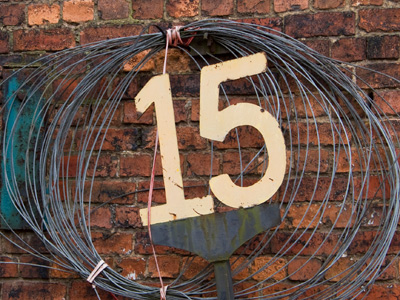3 x 5 = 15.

# 3 Times Table

Learning the three times table involves multiplying numbers by 3, or tripling them. Knowing how to do that helps with all kinds of maths at 11-Plus level and beyond. Even if you get all the questions right the first time you play this quiz, keep on coming back to it as you can improve the time it takes you to answer all the questions. Once you've memorised the three times table it will make multiplying numbers, and so your maths lessons, a lot easier!

There are many things that come in threes. There are three primary colours: red, green and blue. A triangle has three sides. Three-dimensional (3D) means that something has length, width and height. Three is quite an important number.

So, even if you think you already know the three times table, take your time and make sure that you read every question carefully before choosing your answer. Overconfidence can lead to careless mistakes.

1.
3 × 9 = ?
27
24
30
21
You can work this out by first multiplying 3 x 10 and then subtracting 3
2.
3 × 3 = ?
12
3
6
9
3 is the square root of 9
3.
3 × 4 = ?
6
15
9
12
There are 12 months in a year
4.
3 × 6 = ?
18
21
15
24
There are 18 holes on a golf course
5.
3 × 8 = ?
15
27
24
21
Pure gold is 24 carat
6.
3 × 2 = ?
4
6
9
8
Most dice have 6 sides - that's because cubes have 6 faces
7.
3 × 5 = ?
15
18
21
14
To times a number by 5, times it by 10 and then halve your answer
8.
3 × 12 = ?
32
34
36
40
There are 36 inches in a yard
9.
3 × 11 = ?
30
33
36
39
To multiply a number by 11, first times it by 10 and than add the first number
10.
3 × 7 = ?
18
21
24
27
21 used to be the age at which we became adults
Author:  Frank Evans